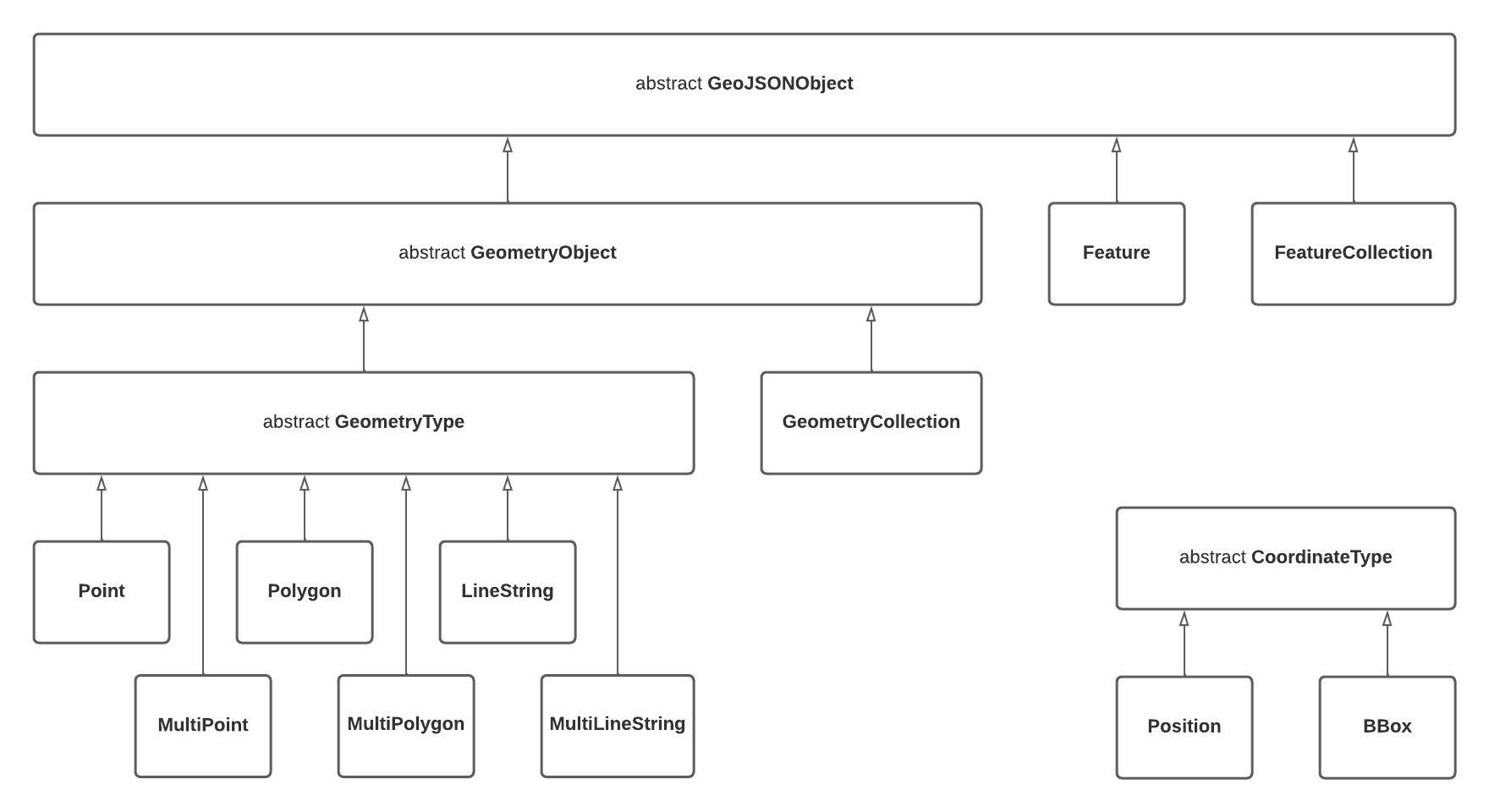# turf.dartTHIS PROJECT IS WORK IN PROCESS

A turf.js-like geospatial analysis library working with GeoJSON, written in pure Dart.

This includes a fully RFC 7946-compliant object-representation and serialization for GeoJSON.

Most of the implementation is a direct translation from turf.js.

## Get started

• Get the Dart tools
• Install the library with `dart pub add turf`
• Import the library in your code and use it. For example:
``````import 'package:turf/helpers.dart';
import 'package:turf/src/line_segment.dart';

Feature<Polygon> poly = Feature<Polygon>(
geometry: Polygon(coordinates: [
[
Position(0, 0),
Position(2, 2),
Position(0, 1),
Position(0, 0),
],
[
Position(0, 0),
Position(1, 1),
Position(0, 1),
Position(0, 0),
],
]),
);

main() {
var total = segmentReduce<int>(
poly,
(previousValue, currentSegment, initialValue, featureIndex,
multiFeatureIndex, geometryIndex, segmentIndex) {
if (previousValue != null) {
previousValue++;
}
return previousValue;
},
0,
combineNestedGeometries: false,
);
print(total);
// total ==  6
}
``````

## GeoJSON Object Model## Notable Design Decisions

• Nested `GeometryCollections` (as described in RFC 7946 section 3.1.8) are not supported which takes a slightly firmer stance than the "should avoid" language in the specification

## Tests and Benchmarks

Tests are run with `dart test` and benchmarks can be run with `dart run benchmark`

Any new benchmarks must be named `*_benchmark.dart` and reside in the `./benchmark` folder.

## Components

### Measurement

• ` ` along
• ` ` area
• `x` bbox
• `x` bboxPolygon
• `x` bearing
• `x` center
• ` ` centerOfMass
• ` ` centroid
• `x` destination
• `x` distance
• ` ` envelope
• ` ` length
• `x` midpoint
• ` ` pointOnFeature
• ` ` polygonTangents
• ` ` pointToLineDistance
• `x` rhumbBearing
• ` ` rhumbDestination
• ` ` rhumbDistance
• ` ` square
• ` ` greatCircle

### Coordinate Mutation

• `x` cleanCoords
• ` ` flip
• ` ` rewind
• ` ` round
• `x` truncate

### Transformation

• ` ` bboxClip
• ` ` bezierSpline
• ` ` buffer
• ` ` circle
• `x` clone - implemented as a member function of each GeoJSONObject
• ` ` concave
• ` ` convex
• ` ` difference
• ` ` dissolve
• ` ` intersect
• ` ` lineOffset
• ` ` polygonSmooth
• ` ` simplify
• ` ` tesselate
• ` ` transformRotate
• ` ` transformTranslate
• ` ` transformScale
• ` ` union
• ` ` voronoi
• `x` polyLineDecode

### MISC

• ` ` ellipse
• ` ` kinks
• ` ` lineArc
• ` ` lineChunk
• ` ` lineIntersect
• ` ` lineOverlap
• `x` lineSegment
• ` ` lineSlice
• ` ` lineSliceAlong
• ` ` lineSplit
• ` ` mask
• `x` nearestPointOnLine
• ` ` sector
• ` ` shortestPath
• ` ` unkinkPolygon

### Random

• ` ` randomPosition
• ` ` randomPoint
• ` ` randomLineString
• ` ` randomPolygon

### Data

• ` ` sample

### Interpolation

• ` ` interpolate
• ` ` isobands
• ` ` isolines
• ` ` planepoint
• ` ` tin

### Joins

• ` ` pointsWithinPolygon
• ` ` tag

### Grids

• ` ` hexGrid
• ` ` pointGrid
• ` ` squareGrid
• ` ` triangleGrid

### Aggregation

• ` ` collect
• ` ` clustersDbscan
• ` ` clustersKmeans

### Booleans

• ` ` booleanClockwise
• ` ` booleanConcave
• ` ` booleanContains
• ` ` booleanCrosses
• ` ` booleanDisjoint
• ` ` booleanEqual
• ` ` booleanIntersects
• ` ` booleanOverlap
• ` ` booleanParallel
• ` ` booleanPointInPolygon
• ` ` booleanPointOnLine
• ` ` booleanWithin

## Libraries

clean_coords.dart
explode
truncate.dart
turf
turf_bbox
turf_bbox_polygon.dart
turf_bearing
turf_center
turf_clusters
turf_destination
turf_distance
turf_extensions
turf_helpers
turf_line_segment
turf_line_to_polygon.dart
turf_meta
turf_midpoint
turf_nearest_point
turf_nearest_point_on_line
turf_polygon_to_line
turf_polyline